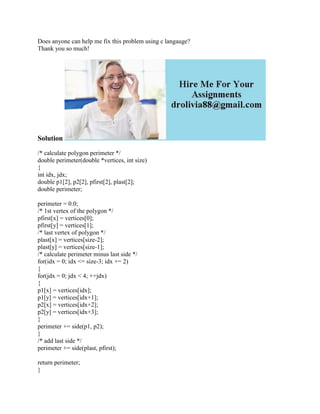Successfully reported this slideshow.

# Does anyone can help me fix this problem using c langauge- Thank you s.docx×

# Does anyone can help me fix this problem using c langauge- Thank you s.docx

Does anyone can help me fix this problem using c langauge?
Thank you so much!
Solution
/* calculate polygon perimeter */
double perimeter(double *vertices, int size)
{
int idx, jdx;
double p1, p2, pfirst, plast;
double perimeter;
perimeter = 0.0;
/* 1st vertex of the polygon */
pfirst[x] = vertices;
pfirst[y] = vertices;
/* last vertex of polygon */
plast[x] = vertices[size-2];
plast[y] = vertices[size-1];
/* calculate perimeter minus last side */
for(idx = 0; idx <= size-3; idx += 2)
{
for(jdx = 0; jdx < 4; ++jdx)
{
p1[x] = vertices[idx];
p1[y] = vertices[idx+1];
p2[x] = vertices[idx+2];
p2[y] = vertices[idx+3];
}
perimeter += side(p1, p2);
}
perimeter += side(plast, pfirst);
return perimeter;
}
vertices means array of vertices stored
.

Does anyone can help me fix this problem using c langauge?
Thank you so much!
Solution
/* calculate polygon perimeter */
double perimeter(double *vertices, int size)
{
int idx, jdx;
double p1, p2, pfirst, plast;
double perimeter;
perimeter = 0.0;
/* 1st vertex of the polygon */
pfirst[x] = vertices;
pfirst[y] = vertices;
/* last vertex of polygon */
plast[x] = vertices[size-2];
plast[y] = vertices[size-1];
/* calculate perimeter minus last side */
for(idx = 0; idx <= size-3; idx += 2)
{
for(jdx = 0; jdx < 4; ++jdx)
{
p1[x] = vertices[idx];
p1[y] = vertices[idx+1];
p2[x] = vertices[idx+2];
p2[y] = vertices[idx+3];
}
perimeter += side(p1, p2);
}